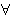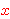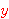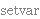Intuitionistic Logic Explorer < Previous   Next > Nearby theorems Mirrors  >  Home  >  ILE Home  >  Th. List  >  wal Unicode version

Syntax Definition wal 1312
 Description: Extend wff definition to include the universal quantifier ('for all').is read "(phi) is true for all." Typically, in its final applicationwould be replaced with a wff containing a (free) occurrence of the variable, for example. In a universe with a finite number of objects, "for all" is equivalent to a big conjunction (AND) with one wff for each possible case of. When the universe is infinite (as with set theory), such a propositional-calculus equivalent is not possible because an infinitely long formula has no meaning, but conceptually the idea is the same.
Hypotheses
Ref Expression
wphvx.walAssertion
Ref Expression
wal